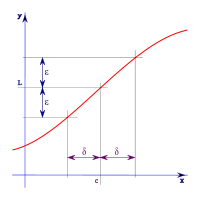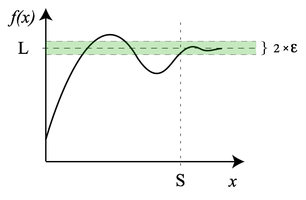# Limit (mathematics)

Limit (mathematics)

In mathematics, the concept of a "limit" is used to describe the value that a function or sequence "approaches" as the input or index approaches some value. The concept of limit allows mathematicians to define a new point from a Cauchy sequence of previously defined points within a complete metric space. Limits are essential to calculus (and mathematical analysis in general) and are used to define continuity, derivatives, and integrals.

The concept of a limit of a sequence is further generalized to the concept of a limit of a topological net, and is closely related to limit and direct limit in category theory.

In formulas, limit is usually abbreviated as lim as in lim(an) = a or represented by the right arrow (→) as in ana.

## Limit of a functionWhenever a point x is within δ units of c, f(x) is within ε units of L. For all x > S, f(x) is within ε of L.

Suppose f(x) is a real-valued function and c is a real number. The expression$\lim_{x \to c}f(x) = L$

means that f(x) can be made to be as close to L as desired by making x sufficiently close to c. In that case, it can be stated that "the limit of f of x, as x approaches c, is L". Note that this statement can be true even if f(c) ≠ L. Indeed, the function f(x) need not even be defined at c.

For example, if$f(x) = \frac{x^2 - 1}{x - 1}$

then f(1) is not defined (see division by zero), yet as x moved arbitrarily close to 1, f(x) correspondingly approaches 2:

 f(0.9) f(0.99) f(0.999) f(1.0) f(1.001) f(1.01) f(1.1) 1.900 1.990 1.999 ⇒ undef ⇐ 2.001 2.010 2.100

Thus, f(x) can be made arbitrarily close to the limit of 2 just by making x sufficiently close to 1.

Augustin-Louis Cauchy in 1821, followed by Karl Weierstrass, formalized the definition of the limit of a function into what became known as the (ε, δ)-definition of limit in the 19th century. The definition uses ε (the lowercase Greek letter epsilon) to represent a small positive number, so that "f(x) becomes arbitrarily close to L" means that f(x) lies in the interval (L - ε, L + ε), which can also be written using absolute value as |f(x) - L| < ε. The statement "x approaches c" then indicates that a positive number δ (the lowercase greek letter delta) exists within either (c - δ, c) or (c, c + δ), which can be expressed with 0 < |x - c| < δ. The first inequality means that the distance between x and c is more than 0 and that x ≠ c, while the second indicates that x is within distance δ of c.

In addition to limits at finite values, functions can also have limits at infinity. For example, consider$f(x) = {2x-1 \over x}$
• f(100) = 1.9900
• f(1000) = 1.9990
• f(10000) = 1.99990

As x becomes extremely large, the value of f(x) approaches 2, and the value of f(x) can be made as close to 2 as one could wish just by picking x sufficiently large. In this case, the limit of f(x) as x approaches infinity is 2. In mathematical notation,$\lim_{x \to \infty} f(x) = 2.$

## Limit of a sequence

Consider the following sequence: 1.79, 1.799, 1.7999,... It can be observed that the numbers are "approaching" 1.8, the limit of the sequence.

Formally, suppose x1, x2, ... is a sequence of real numbers. It can be stated that the real number L is the limit of this sequence, namely:$\lim_{n \to \infty} x_n = L$

to mean

For every real number ε > 0, there exists a natural number n0 such that for all n > n0, |xn − L| < ε.

Intuitively, this means that eventually all elements of the sequence get as close as needed to the limit, since the absolute value |xn − L| is the distance between xn and L. Not every sequence has a limit; if it does, it is called convergent, and if it does not, it is divergent. One can show that a convergent sequence has only one limit.

The limit of a sequence and the limit of a function are closely related. On one hand, the limit of a sequence is simply the limit at infinity of a function defined on natural numbers. On the other hand, a limit of a function f at x, if it exists, is the same as the limit of the sequence f(an) where an is any arbitrary sequence whose limit is x, and where an is never equal to x. Note that one such sequence would be x + 1/n.

## Convergence and fixed point

A formal definition of convergence can be stated as follows. Suppose pn as n goes from 0 to$\infty$ is a sequence that converges to a fixed point p, with${p}_{n} \neq 0$ for all n. If positive constants λ and α exist with$\lim_{n \rightarrow \infty } \frac{ \left | { p}_{n+1 } -p \right | }{ { \left | { p}_{n }-p \right | }^{ \alpha} } =\lambda$

then pn as n goes from 0 to$\infty$ converges to p of order α, with asymptotic error constant λ

Given a function f(x) = x with a fixed point p, there is a nice checklist for checking the convergence of p.

1) First check that p is indeed a fixed point:
f(p) = p
2) Check for linear convergence. Start by finding$\left | f^\prime (p) \right |$. If....$\left | f^\prime (p) \right | \in (0,1)$ then there is linear convergence$\left | f^\prime (p) \right | > 1$ series diverges$\left | f^\prime (p) \right | =0$ then there is at least linear convergence and maybe something better, the expression should be checked for quadratic convergence
3) If it is found that there is something better than linear the expression should be checked for quadratic convergence. Start by finding$\left | f^{\prime\prime} (p) \right |$ If....$\left | f^{\prime\prime} (p) \right | \neq 0$ then there is quadratic convergence provided that$f^{\prime\prime} (p)$ is continuous$\left | f^{\prime\prime} (p) \right | = 0$ then there is something even better than quadratic convergence$\left | f^{\prime\prime} (p) \right |$ does not exist then there is convergence that is better than linear but still not quadratic



## Topological net

All of the above notions of limit can be unified and generalized to arbitrary topological spaces by introducing topological nets and defining their limits.

An alternative is the concept of limit for filters on topological spaces.

Wikimedia Foundation. 2010.

### См. также в других словарях:

• Limit — A limit can be: * Limit (mathematics), including: ** Limit of a function ** Limit of a sequence ** One sided limit ** Limit superior and limit inferior ** Limit of a net ** Limit point ** Limit (category theory) * A constraint (mathematical,… …   Wikipedia

• Mathematics and art — have a long historical relationship. The ancient Egyptians and ancient Greeks knew about the golden ratio, regarded as an aesthetically pleasing ratio, and incorporated it into the design of monuments including the Great Pyramid, the Parthenon …   Wikipedia

• Mathematics (UIL) — Mathematics (sometimes referred to as General Math, to distinguish it from other mathematics related events) is one of several academic events sanctioned by the University Interscholastic League. It is also a competition held by the Texas Math… …   Wikipedia

• Limit of a function — x 1 0.841471 0.1 0.998334 0.01 0.999983 Although the function (sin x)/x is not defined at zero, as x becomes closer and closer to zero, (sin x)/x becomes arbitrarily close to 1. It is said that the limit of (sin x)/x as x approache …   Wikipedia

• mathematics — /math euh mat iks/, n. 1. (used with a sing. v.) the systematic treatment of magnitude, relationships between figures and forms, and relations between quantities expressed symbolically. 2. (used with a sing. or pl. v.) mathematical procedures,… …   Universalium

• Mathematics and Physical Sciences — ▪ 2003 Introduction Mathematics       Mathematics in 2002 was marked by two discoveries in number theory. The first may have practical implications; the second satisfied a 150 year old curiosity.       Computer scientist Manindra Agrawal of the… …   Universalium

• mathematics, foundations of — Scientific inquiry into the nature of mathematical theories and the scope of mathematical methods. It began with Euclid s Elements as an inquiry into the logical and philosophical basis of mathematics in essence, whether the axioms of any system… …   Universalium

• Limit superior and limit inferior — In mathematics, the limit inferior (also called infimum limit, liminf, inferior limit, lower limit, or inner limit) and limit superior (also called supremum limit, limsup, superior limit, upper limit, or outer limit) of a sequence can be thought… …   Wikipedia

• Limit (category theory) — In category theory, a branch of mathematics, the abstract notion of a limit captures the essential properties of universal constructions such as products and inverse limits. The dual notion of a colimit generalizes constructions such as disjoint… …   Wikipedia

• Limit-cycle — Attracting limit cycle and its Poincaré map In mathematics, in the area of dynamical systems, a limit cycle on a plane or a two dimensional manifold is a closed trajectory in phase space having the property that at least one other trajectory… …   Wikipedia

### Поделиться ссылкой на выделенное

##### Прямая ссылка:
Нажмите правой клавишей мыши и выберите «Копировать ссылку»